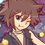# Any ideas on solving this equation? (help meh!)

Solve the equation in real number.

$\sqrt{2+\sqrt{2+\sqrt{2+x}}} + \sqrt{3}\sqrt{2-\sqrt{2+\sqrt{2+x}}} = 2x$

I've got an idea that $0 \leq x \leq 2$, but I can't continue further.

Help me!Note by Samuraiwarm Tsunayoshi
6 years ago

This discussion board is a place to discuss our Daily Challenges and the math and science related to those challenges. Explanations are more than just a solution — they should explain the steps and thinking strategies that you used to obtain the solution. Comments should further the discussion of math and science.

When posting on Brilliant:

• Use the emojis to react to an explanation, whether you're congratulating a job well done , or just really confused .
• Ask specific questions about the challenge or the steps in somebody's explanation. Well-posed questions can add a lot to the discussion, but posting "I don't understand!" doesn't help anyone.
• Try to contribute something new to the discussion, whether it is an extension, generalization or other idea related to the challenge.
• Stay on topic — we're all here to learn more about math and science, not to hear about your favorite get-rich-quick scheme or current world events.

MarkdownAppears as
*italics* or _italics_ italics
**bold** or __bold__ bold
- bulleted- list
• bulleted
• list
1. numbered2. list
1. numbered
2. list
Note: you must add a full line of space before and after lists for them to show up correctly
paragraph 1paragraph 2

paragraph 1

paragraph 2

[example link](https://brilliant.org)example link
> This is a quote
This is a quote
    # I indented these lines
# 4 spaces, and now they show
# up as a code block.

print "hello world"
# I indented these lines
# 4 spaces, and now they show
# up as a code block.

print "hello world"
MathAppears as
Remember to wrap math in $$ ... $$ or $ ... $ to ensure proper formatting.
2 \times 3 $2 \times 3$
2^{34} $2^{34}$
a_{i-1} $a_{i-1}$
\frac{2}{3} $\frac{2}{3}$
\sqrt{2} $\sqrt{2}$
\sum_{i=1}^3 $\sum_{i=1}^3$
\sin \theta $\sin \theta$
\boxed{123} $\boxed{123}$

## Comments

Sort by:

Top Newest

Substitute $x=2\cos(\theta)$

$\therefore$ $\sqrt{2+\sqrt{2+\sqrt{2+2\cos(\theta)}}} + \sqrt{3}\sqrt{2-\sqrt{2+\sqrt{2+2\cos(\theta)}}}= 4\cos(\theta)$

Now, $2+2\cos(\theta)= 2(1+\cos(\theta))= 4cos^{2}(\theta/2)$

Hence are equation becomes: $\sqrt{2+\sqrt{2+2|\cos(\theta/2)|}}+ \sqrt{3}\sqrt{2-\sqrt{2+2|\cos(\theta/2)|}}=4\cos(\theta)$

Repeat this process till you eliminate the radicals( it will take you two more steps).

Note: In the last step you will have to use $1-\cos(\theta/4)=2\sin^{2}(\theta/8)$

So finally we arrive at : $2|\cos(\theta/8)|+2\sqrt{3}|\sin(\theta/8)|=4\cos(\theta)$

$\therefore$ $\dfrac{1}{2}|\cos(\theta/8)|+ \dfrac{\sqrt{3}}{2}|\sin(\theta/8)|=\cos(\theta)$

$\text{CASE 1: When \theta lies in the first quadrant}$

$\bullet$ $\cos(\theta/8-\pi/3)=cos(\theta)$

$\text{CASE 2: When theta lies in the second quadrant}$

$\bullet$ $-\cos(\theta/8+\pi/3)= cos(\theta)$

$\text{CASE 3: When theta lies in the third quadrant}$

$\bullet$ $-\cos(\theta/8-\pi/3)=cos(\theta)$

$\text{CASE 4: When theta lies in the fourth quadrant}$

$\bullet$ $\cos(\theta/8+\pi/3)= cos(\theta)$

Solve this general equation for $\theta$, take the union of all cases and plug the permissible values in $x=2\cos(\theta)$, to find your desired solutions.

Feel free to ask if you have a doubt at any point.

- 6 years ago

Log in to reply

Great work!!

However, you will need to be careful, that $\sqrt{ \cos^2 \theta } = | \cos \theta |$. So, after the last step, proceed with caution.

Note that in the second line, the RHS should be $4 \cos ^2 ( \theta / 2 )$.

Staff - 6 years ago

Log in to reply

Oh yes, I had completely forgotten that, Thanks a ton sir, for your inputs!

- 6 years ago

Log in to reply

Sir, but $0\leq x \leq 2$, Hence $0\leq y \leq 1$, where $y=\cos(\theta)$ So the modulus here has no importance, right?

- 6 years ago

Log in to reply

Firstly, I disagree with Samuraiwarm's claim that $0 \leq x \leq 2$. Looking at the domain of the most nested square root, we get $x +2 \geq 0$. We need to look further at $\sqrt{ 2 - \sqrt{ 2 + \sqrt{ 2 + x } } }$ (requires some work) to be able to conclude that $x \leq 2$.

Putting this together, we actually have $-2 \leq x \leq 2$. So, you should verify the assumptions, especially when it's phrased as "I have an idea that this might work". It just might be possible that we have negative solutions.

Secondly, (for sake of argument) suppose you found that $\theta = \frac{ 7 \pi } { 4}$ was a solution. Is this truly a solution? The issue arises because even though $\cos \theta > 0$, we actually end up with $\cos \frac{ \theta } { 2} < 0$. As such, we actually have $\sqrt{ 2 + 2 \cos \theta } = - \cos \frac{ \theta} {2} \neq \cos \frac{ \theta}{2}$ IE we need to take the negative square root.

Staff - 6 years ago

Log in to reply

Actually i thought it was given as a constraint along with the equation. Sir i've made the necessary changes. Can you check it once, please?

- 6 years ago

Log in to reply

Consider LHS; both sides are square roots, which is always non-negative. That means RHS is always non-negative as well.

Log in to reply

Oh, I missed that. Thanks!

Staff - 6 years ago

Log in to reply

Try some trigonometry.

- 6 years ago

Log in to reply

I haven't been able to work on this, Sir, But the range $0\le x\le2$ made me think of the substitution $x=X+1$. Therefore, $X\in[-1,1]$ which immediately reminds one of $\sin\theta$ or $\cos\theta$.

- 6 years ago

Log in to reply

I think it would be better if you would cosider x=2X rather than x=X+1 and then you can use trigo.

- 6 years ago

Log in to reply

Perhaps. Like I said, I haven't tried this at all and won't be able to till around late evening.

- 6 years ago

Log in to reply

Hint: Conjugates.

Staff - 6 years ago

Log in to reply

×

Problem Loading...

Note Loading...

Set Loading...